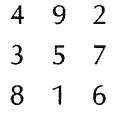# magic square

Also found in: Dictionary, Thesaurus, Wikipedia.

## magic square,

a square divided into parts with letters or numbers inscribed therein that, whether combined vertically, horizontally, or diagonally, form the same sum or the same word. In ancient times such squares were thought to have magic properties, perhaps connected with the stars. Magic squares have been found in such widely divergent cultures as ancient China, Egypt, and India, as well as W Europe. Example:### Bibliography

See W. S. Andrews, Magic Squares and Cubes (2d ed. 1917, repr. 1960).

## square, magic:

see magic squaremagic square,
a square divided into parts with letters or numbers inscribed therein that, whether combined vertically, horizontally, or diagonally, form the same sum or the same word.
.
The following article is from The Great Soviet Encyclopedia (1979). It might be outdated or ideologically biased.

## Magic Square

a square divided into an equal number n of columns and rows with the resulting cells containing the first n2 natural numbers whose sum in each column, each row, and the two main diagonals is the same [being equal, as can be shown, to (½) n(n2 + 1)]. It has been proved that a magic square can be constructed for any n beginning with n = 3. Figure 1, a shows magic squares for n = 3 and n = 4. There exist magic squares that satisfy a number of additional conditions, as for example, a magic square with 64 cells (see Figure 1, b), which can be divided into four smaller squares each containing 16 cells such that the sum of the numbers of any row, any column, or the main diagonals is the same (130). Magic squares were used as talismans in India and some other countries. The construction of magic squares is a classical example of mathematical games and puzzles.Figure 1

### REFERENCE

Postnikov, M. M. Magicheskie kvadraty. Moscow, 1964.

## magic square

[′maj·ik ′skwer]
(mathematics)
A square array of integers where the sum of the entries of each row, each column, and each diagonal is the same.
A square array of integers where the sum of the entries in each row and each column (but not necessarily each diagonal) is the same. Also known as semimagic square.
McGraw-Hill Dictionary of Scientific & Technical Terms, 6E, Copyright © 2003 by The McGraw-Hill Companies, Inc.
References in periodicals archive ?
answers WHO WHAT WHERE WHEN: Nathanael West; Canoeing; Off the west coast of Scotland; December, 1967 REMEMBER WHEN: 1967 IMPOSSIPUZZLES: Magic Square has 16 numbers, 5 to 20.
This square is a variation of magic squares called "Most Perfect" by England's Kathleen Ollerenshaw (1912=2014).
We continued to work on magic squares and even some short exercises in logic (which fifth graders find much more appealing if it is called algebra).
Sallows found that for columns, rows and diagonals totaling less than 200, French has only one such magic square while English has more than seven.
It is a fact that for all n equaling 3 or greater our method will always produce an n x n magic square with constant n[sup.2] at least on rows, columns, and the two main diagonals.
Magicians are no doubt familiar with the old puzzle of placing the 16 face cards of a deck into a 4x4 magic square. An early reference is Jacques Ozanam's 1694 edition Recreations Mathematique et Physiques.
In today's square that magic total happens to be exactly one third of the square of the smallest number in the array, and we have the smallest magic square for which this can be so.
The story goes that the first magic square ever seen was in 2800BC.
1 Cecil Parkinson resigned from the cabinet 2 Gerry Adams replaced Ruairi O'Bradaigh as the leader of Sinn Fein 3 Ex-President Ford made his acting debut in the soap opera Dynasty 4 Stern magazine published the fake "Hitler Diaries" impossipuzzles Today we have a magic square. That's an array of 16 consecutive positive numbers in square formation, chessboard style.
Of the many symbolic references in the etching, one often cited is the magic square as a Jovian device used to counteract the unfavorable influence of Saturn.
1 Prince Charles was born 2 Harry Truman was elected US President 3 The railways were nationalised 4 Communists seized power in Czechoslovakia impossipuzzles We have a magic square. It's a sequence of 16 consecutive positive numbers, arranged in a square chessboard formation, so that each column, each row, and each diagonal will add up to the same magic total.
ANSWERS V 1 P 2 K 3 G 4 T 5 L 6 S 7 W 8 M 9 Q 10 A 11 R 12 J 13 U 14 E 15 N 16 O 17 X 18 B 19 Z 20 Y 21 F 22 C 23 D 24 H 25 I 26 MAGIC SQUARE: chat; hype; apes; test.

Site: Follow: Share:
Open / Close